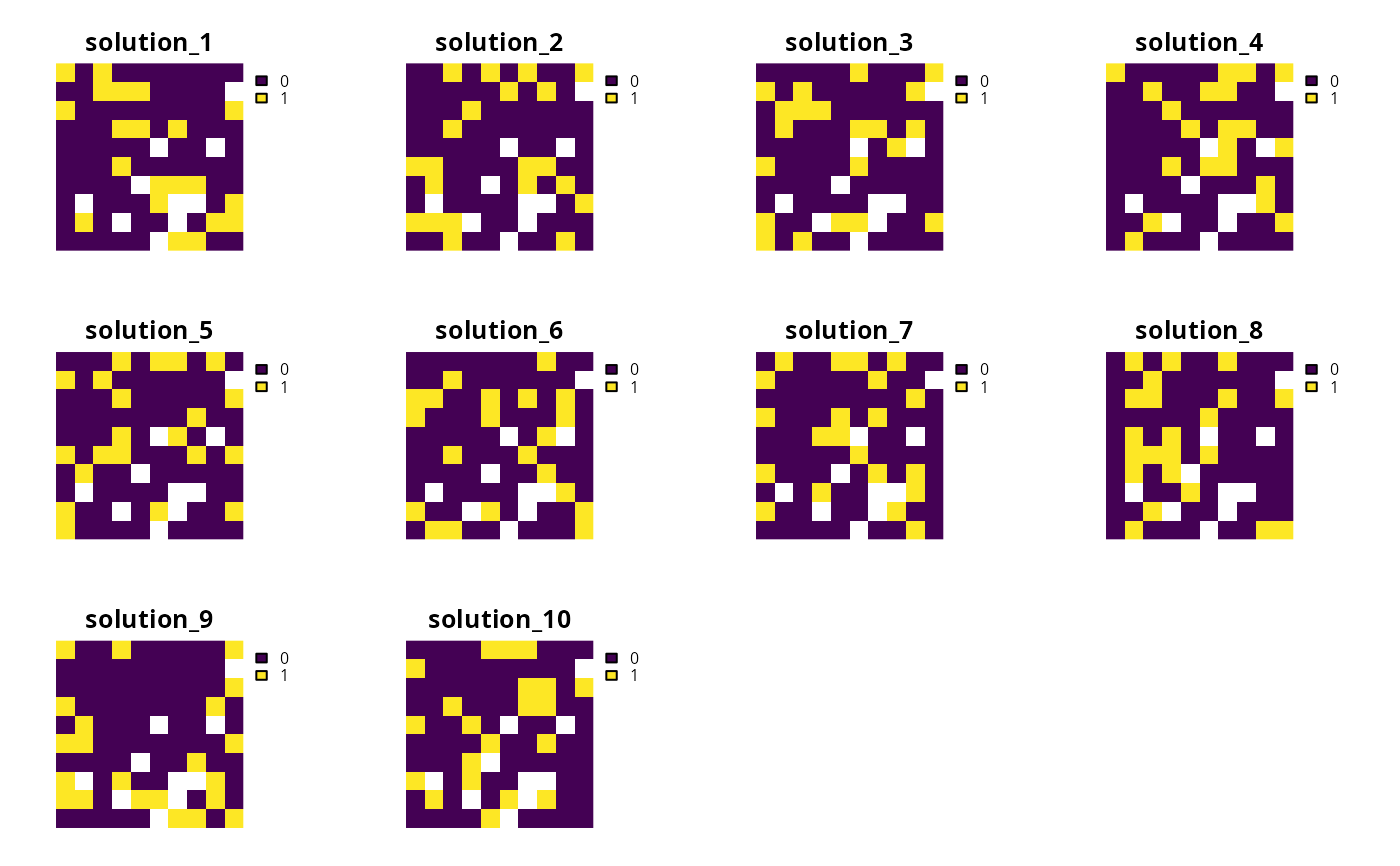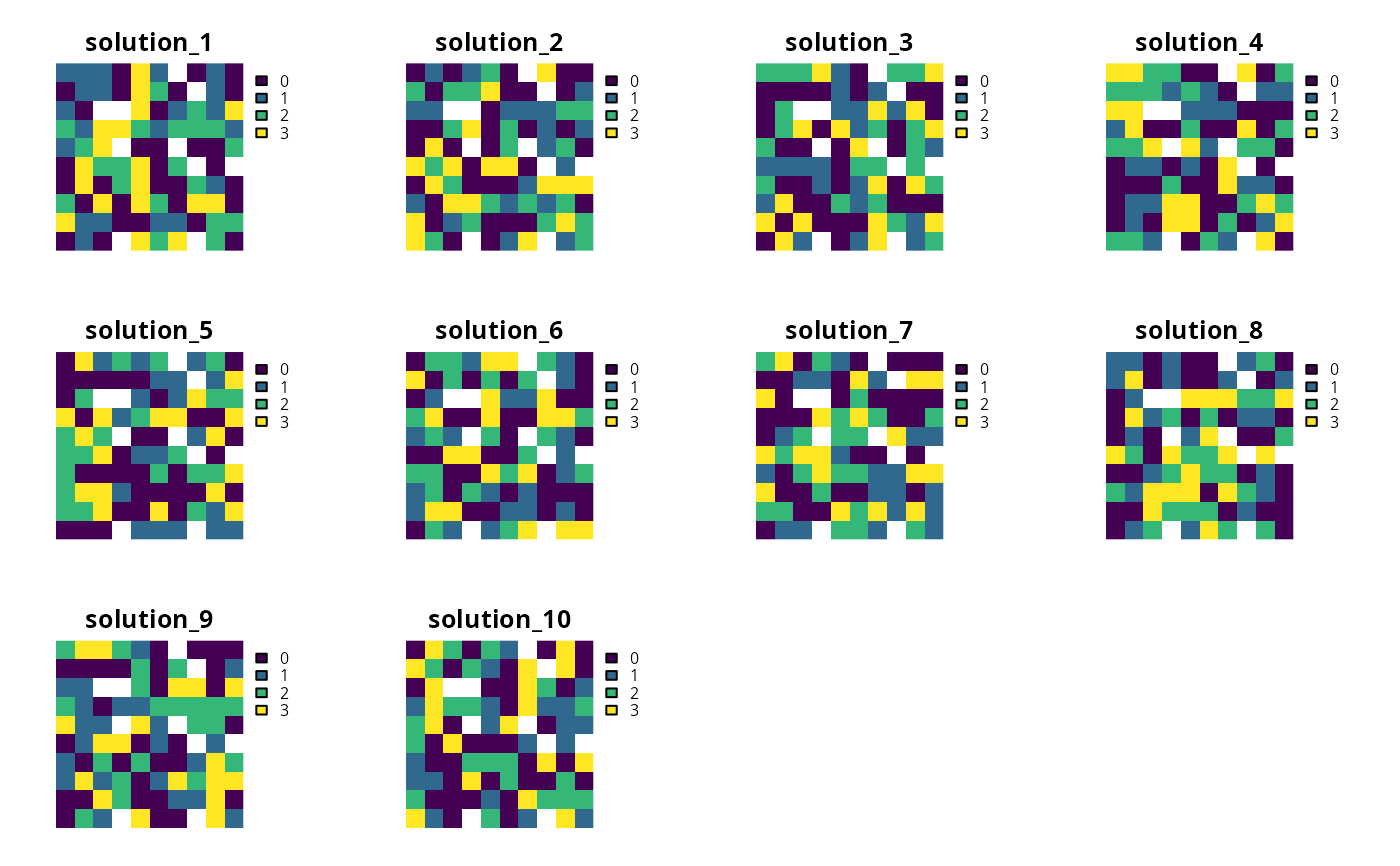Generate a portfolio of solutions for a conservation planning problem by randomly reordering the data prior to solving the problem. This is recommended as a replacement for add_top_portfolio() when the Gurobi software is not available.

## Usage

add_shuffle_portfolio(
x,
number_solutions = 10,
remove_duplicates = TRUE
)

## Arguments

x

problem() object.

number_solutions

integer number of attempts to generate different solutions. Defaults to 10.

integer number of threads to use for the generating the solution portfolio. Defaults to 1.

remove_duplicates

logical should duplicate solutions be removed? Defaults to TRUE.

## Value

An updated problem() object with the portfolio added to it.

## Details

This strategy for generating a portfolio of solutions often results in different solutions, depending on optimality gap, but may return duplicate solutions. In general, this strategy is most effective when problems are quick to solve and multiple threads are available for solving each problem separately.

See portfolios for an overview of all functions for adding a portfolio.

Other portfolios: add_cuts_portfolio(), add_extra_portfolio(), add_gap_portfolio(), add_top_portfolio()

## Examples

# \dontrun{
# set seed for reproducibility
set.seed(500)

sim_pu_raster <- get_sim_pu_raster()
sim_features <- get_sim_features()
sim_zones_pu_raster <- get_sim_zones_pu_raster()
sim_zones_features <- get_sim_zones_features()

# create minimal problem with shuffle portfolio
p1 <-
problem(sim_pu_raster, sim_features) %>%
add_default_solver(gap = 0.2, verbose = FALSE)

# solve problem and generate 10 solutions within 20% of optimality
s1 <- solve(p1)

# convert portfolio into a multi-layer raster
s1 <- terra::rast(s1)

# print number of solutions found
print(terra::nlyr(s1))
#>  10

# plot solutions in portfolio
plot(s1, axes = FALSE)# build multi-zone conservation problem with shuffle portfolio
p2 <-
problem(sim_zones_pu_raster, sim_zones_features) %>%
add_relative_targets(matrix(runif(15, 0.1, 0.2), nrow = 5, ncol = 3)) %>%
add_default_solver(gap = 0.2, verbose = FALSE)

# solve the problem
s2 <- solve(p2)

# convert each solution in the portfolio into a single category layer
s2 <- terra::rast(lapply(s2, category_layer))

# print number of solutions found
print(terra::nlyr(s2))
#>  10

# plot solutions in portfolio
plot(s2, axes = FALSE)# }